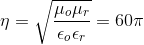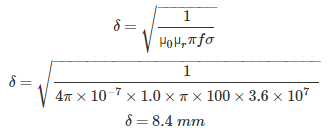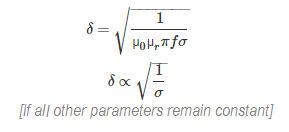# MCQs on Electromagnetic Fields

##### Page 12 of 20. Go to page 1 2 3 4 5 6 7 8 9 10 11 12 13 14 15 16 17 18 19 20
01․ In a dielectric material an applied field in x direction Ex = 5 v/m gives a polarization of px = 3/10π ux nc/m2. The susceptibility of the material is
Zero.
Infinity.
4.32.
2.16.

P = εor – 1) E. Susceptibility = εr – 1 = P/(εo. E).

02․ A pure inductor is connected across a voltage source. Find the active power drawn by the inductor?
0
maximum
either 1 or 2
none of the above

Active power P = VI cosφ Where V = voltage cross inductor I = current through inductor φ = phase angle between voltage and current When a pure inductor is connected across a voltage source, current lags the voltage by exactly 90°. Therefore, P =VI cos 90 P = 0

03․ In free space E (z, t) = 103 sin(ωt - βz) uy v/m. What is the value of H (z, t)
(103/377) sin(ωt - β z)(ux) A/m.
(103/377) cos(ωt - β z)(-ux) A/m.
(103/377) sin(ωt - β z)(-ux) A/m.
None of above.

Direction of wave propagation (+z). So E × H must be (+z) direction. Therefore E × H must be – ux Now Ey /Hx = ηo = 377 (As free space) Hx = 103/377 (A/m).

04․ In a lossless medium the intrinsic impedance η = 60π and μr = 1. The relative dielectric constant εr shall be
4.
2.
1.
8.

For free space ηo; {√(μ/ε) = 120π for any lossless medium with μr= 1}So, εr = 4.

05․ A plane wave magnetic field is represented by Bx = cos(y – ct). The electric and magnetic fields will be zero in the direction
Ex = Ey = 0, Bx = By = 0.
Ey = Ez = 0, By = Bz = 0.
Ex = Ey = 0, By = Bz = 0.
None of these.

Wave propagating in (+ y) direction. H in (+ x) direction Then E will be in (+ z) direction. So, Ex = Ey = 0 and By = Bz = 0.

06․ What is the value of skin depth as 100 Hz in a material having μr = 1.0 and & σ = 3.60 × 107 s/m
2.4 mm.
4 mm.
8.4 mm.
10 mm.

µ0 = 4π × 10-7 Skin depth of a material is given as,07․ The frequency of the power wave associated with an electromagnetic wave having field as E = e-z/δ cos(ω - zδ) is given by
ω.
ω/4π.
ω/2π.
ω / π.

Here E = e - zδ cos(ω t – z/δ) Where, ω is frequency in radian of E. It is same the radian frequency of the associated H wave. The frequency of power wave is double the corresponding frequency of E or H wave. Thus the radian frequency of 2ω and cyclic frequency = 2ω/2π = ω / π.

08․ Skin depth is proportional to
Frequency.
√(σ).
1/√σ.
Permeability.

Skin depth is given asWhere, σ = conductivity in mho/m

09․ A plane wave in a homogeneous medium has E = 50 sin(108t + 2z) uy V/m. What is the direction of wave propagation
– z direction.
z direction.
– y direction.
y direction.

A wave E = 50 sin(108 t – 2z) uy has vector component of E in y direction and it travels in z direction. The given wave with +2z shall move in the negative z direction. But here it will move in the negative direction.

10․ If n is the polarization vector and k is the direction of propagation plane electromagnetic wave, then
n = K.
n = - k.
n × k = 0.
n. k = 0.

The polarization vector and direction of travel are perpendicular to each other. So, dot product of these two vectors will be zero.

<<<1011121314>>>